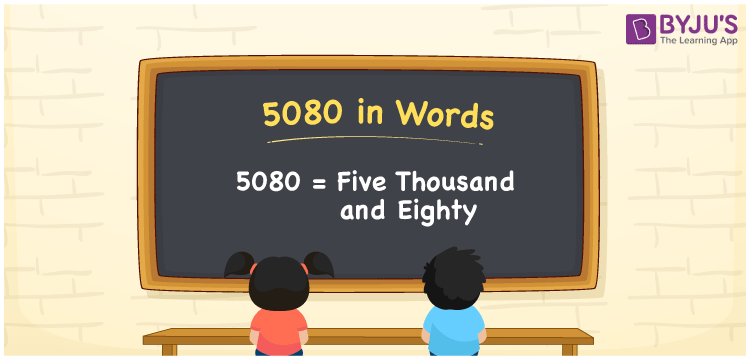# 5080 in words

5080 in words is written as Five Thousand and Eighty. 5080 represents the count or value. The article on Counting Numbers can give you an idea about count or counting. The number 5080 is used in expressions that relate to money, distance, length, year and others. Let us consider an example for 5080. “The grocery for the month of February costs Five Thousand and Eighty Rupees” Another example, “A bikers team completed a road trip of Five Thousand Eighty kilometers.”

 5080 in words Five Thousand and Eighty Five Thousand and Eighty in Numbers 5080

## 5080 in English Words## How to Write 5080 in Words?

We can convert 5080 to words using a place value chart. The number 5080 has 4 digits, so let’s make a chart that shows the place value up to 4 digits.

 Thousands Hundreds Tens Ones 5 0 8 0

Thus, we can write the expanded form as:

5 × Thousand + 0 × Hundred + 8 × Ten + 0 × One

= 5 × 1000 + 0 × 100 + 8 × 10 + 0 × 1

= 5080

= Five Thousand and Eighty.

5080 is the natural number that is succeeded by 5079 and preceded by 5081.

5080 in words – Five Thousand and Eighty.

Is 5080 an odd number? – No.

Is 5080 an even number? – Yes.

Is 5080 a perfect square number? – No.

Is 5080 a perfect cube number? – No.

Is 5080 a prime number? – No.

Is 5080 a composite number? – Yes.

## Solved Example

1. Write the number 5080 in expanded form

Solution: 5 × 1000 + 0 × 100 + 8 × 10 + 0 × 1

We can write 5080 = 5000 + 000 + 80 + 0

= 5 × 1000 + 0 × 100 + 8 × 10 + 0 × 1

## Frequently Asked Questions on 5080 in words

Q1

### How to write 5080 in words?

5080 in words is written as Five Thousand and Eighty.
Q2

### State if True or False. 5080 is divisible by 2?

True. 5080 is divisible by 2.
Q3

### Is 5080 a perfect cube number?

No. 5080 is not a perfect cube number.